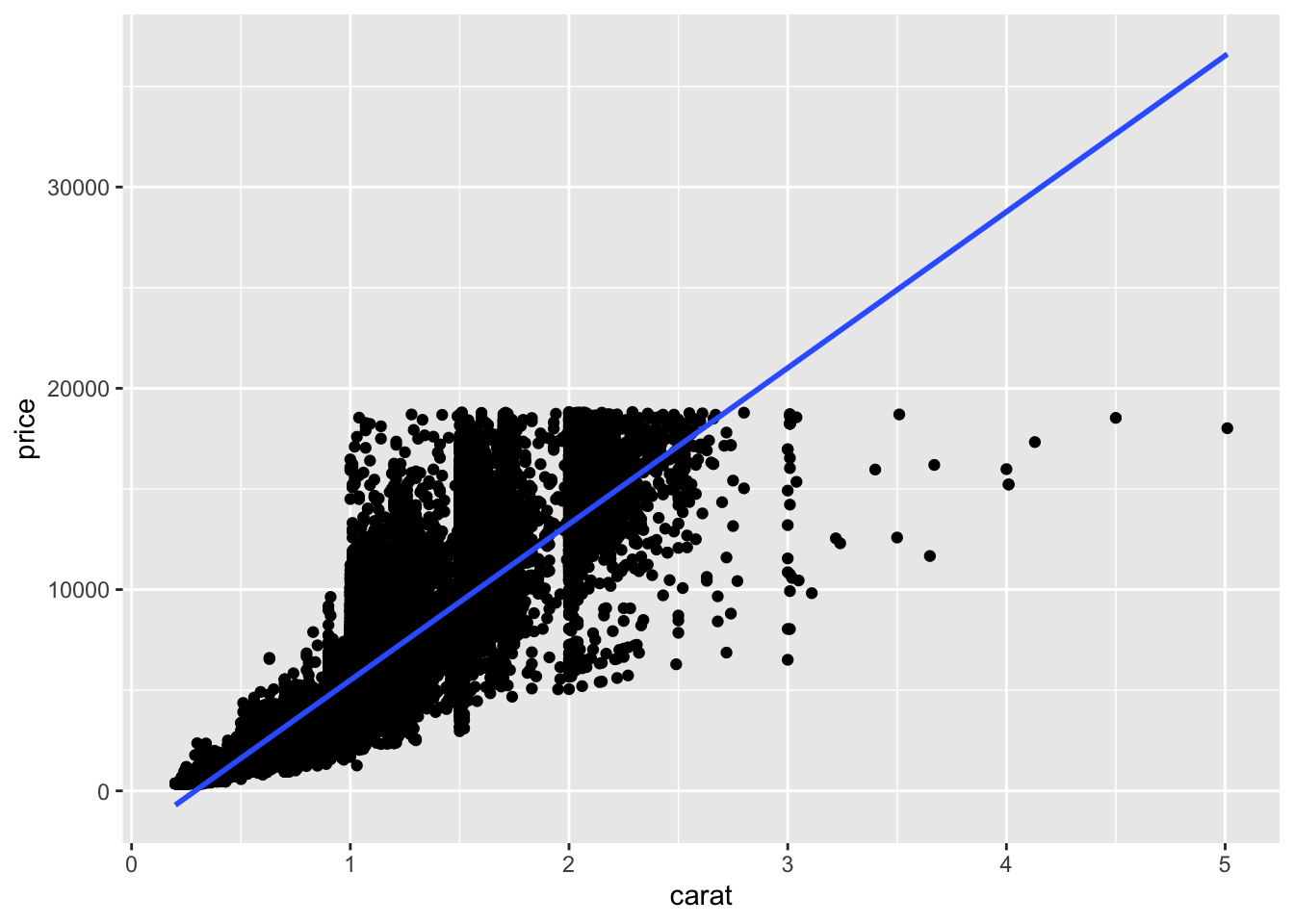Author

Published

October 12, 2022

# Required Packages & Data

Load all the required packages we will use (note I have installed them already into the cloud project) by running (clicking the green play button) the chunk below:

library(tidyverse) # your friend and mine
library(broom) # for tidy regression
library(modelsummary) # for nice regression tables
library(infer) # for simulating inference

What is the effect of a diamond’s carat size on its price?

We will use the diamonds example dataset that comes bundled with ggplot, automatically loaded with tidyverse. To make it an explicit tibble in our workspace, run the code below.

diamonds <- diamonds

Note I am calling the tibble diamonds, feel free to name it whatever you like, but I will assume in my code chunks below that you are calling it diamonds - change as appropriate.

## Question 1

Just to see what we’re looking at, make a scatterplot using ggplot(), with x as carat and y as price, and add a regression line.

# type your code below in this chunk
ggplot(data = diamonds)+
aes(x = carat,
y = price)+
geom_point()+
geom_smooth(method = "lm")
geom_smooth() using formula 'y ~ x'# Regression

## Question 2

Suppose we want to estimate the following relationship:

$\text{Price}_i = \beta_0+\beta_1 \, \text{carat}_i+u_i$

Run a regression of price on carat using lm() and get a summary() of it. Be sure to save your regression model as an object, we’ll need it later.

# type your code below in this chunk
reg <- lm(price ~ carat, data = diamonds)

summary(reg)

Call:
lm(formula = price ~ carat, data = diamonds)

Residuals:
Min       1Q   Median       3Q      Max
-18585.3   -804.8    -18.9    537.4  12731.7

Coefficients:
Estimate Std. Error t value Pr(>|t|)
(Intercept) -2256.36      13.06  -172.8   <2e-16 ***
carat        7756.43      14.07   551.4   <2e-16 ***
---
Signif. codes:  0 '***' 0.001 '**' 0.01 '*' 0.05 '.' 0.1 ' ' 1

Residual standard error: 1549 on 53938 degrees of freedom
Multiple R-squared:  0.8493,    Adjusted R-squared:  0.8493
F-statistic: 3.041e+05 on 1 and 53938 DF,  p-value: < 2.2e-16

## Question 3

### Part A

Write out the estimated regression equation.

$\widehat{price}_i=−2256.36+7756.43 \, \text{carat}_i$

### Part B

Make a regression table of the output (using the modelsummary package).

# type your code below in this chunk
modelsummary(models = list("Price" = reg),
fmt = 2, # round to 2 decimals
output = "html",
coef_rename = c("(Intercept)" = "Constant",
"carat" = "Carat"),
gof_map = list(
list("raw" = "nobs", "clean" = "n", "fmt" = 0),
list("raw" = "r.squared", "clean" = "R<sup>2</sup>", "fmt" = 2),
list("raw" = "rmse", "clean" = "SER", "fmt" = 2)
),
escape = FALSE,
stars = c('*' = .1, '**' = .05, '***' = 0.01)
)
Price
Constant −2256.36***
(13.06)
Carat 7756.43***
(14.07)
n 53940
R2 0.85
SER 1548.53
* p < 0.1, ** p < 0.05, *** p < 0.01

### Part C

What is the estimated $$\hat{\beta}_1$$ for this model? What does it mean in terms of the context of our question?

### Part C

Now instead of get_confidence_interval(), pipe your object from Part A into visualize() to see the sampling distribution of $$\hat{\beta}_1$$ we simulated. We can add + shade_ci(endpoints = ...) setting the argument equal to whatever our object containing the confidence interval from Question 2 Part D (I have it named here as ci_endpoints).

boot %>%
visualize()+
shade_ci(endpoints = ci_endpoints)